# Set-Operations with Overlay¶

When working with multiple spatial datasets – especially multiple polygon or line datasets – users often wish to create new shapes based on places where those datasets overlap (or don’t overlap). These manipulations are often referred using the language of sets – intersections, unions, and differences. These types of operations are made available in the geopandas library through the `overlay()` method.

The basic idea is demonstrated by the graphic below but keep in mind that overlays operate at the DataFrame level, not on individual geometries, and the properties from both are retained. In effect, for every shape in the left `GeoDataFrame`, this operation is executed against every other shape in the right `GeoDataFrame`: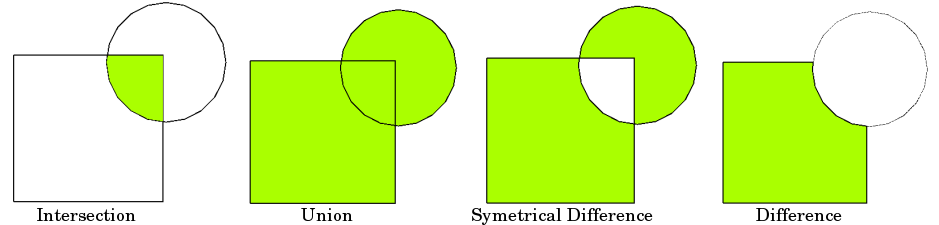Source: QGIS Documentation

Note

Note to users familiar with the shapely library: `overlay()` can be thought of as offering versions of the standard shapely set-operations that deal with the complexities of applying set operations to two GeoSeries. The standard shapely set-operations are also available as `GeoSeries` methods.

## The different Overlay operations¶

First, we create some example data:

```In : from shapely.geometry import Polygon

In : polys1 = geopandas.GeoSeries([Polygon([(0,0), (2,0), (2,2), (0,2)]),
...:                               Polygon([(2,2), (4,2), (4,4), (2,4)])])
...:

In : polys2 = geopandas.GeoSeries([Polygon([(1,1), (3,1), (3,3), (1,3)]),
...:                               Polygon([(3,3), (5,3), (5,5), (3,5)])])
...:

In : df1 = geopandas.GeoDataFrame({'geometry': polys1, 'df1':[1,2]})

In : df2 = geopandas.GeoDataFrame({'geometry': polys2, 'df2':[1,2]})
```

These two GeoDataFrames have some overlapping areas:

```In : ax = df1.plot(color='red');

In : df2.plot(ax=ax, color='green', alpha=0.5);
```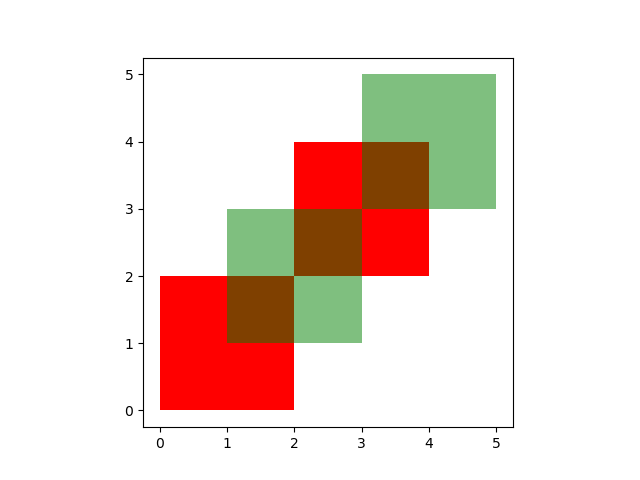We illustrate the different overlay modes with the above example. The `overlay()` method will determine the set of all individual geometries from overlaying the two input GeoDataFrames. This result covers the area covered by the two input GeoDataFrames, and also preserves all unique regions defined by the combined boundaries of the two GeoDataFrames.

Note

For historical reasons, the overlay method is also available as a top-level function `overlay()`. It is recommended to use the method as the function may be deprecated in the future.

When using `how='union'`, all those possible geometries are returned:

```In : res_union = df1.overlay(df2, how='union')

In : res_union
Out:
df1  df2                                           geometry
0  1.0  1.0  POLYGON ((2.00000 2.00000, 2.00000 1.00000, 1....
1  2.0  1.0  POLYGON ((2.00000 2.00000, 2.00000 3.00000, 3....
2  2.0  2.0  POLYGON ((4.00000 4.00000, 4.00000 3.00000, 3....
3  1.0  NaN  POLYGON ((2.00000 0.00000, 0.00000 0.00000, 0....
4  2.0  NaN  MULTIPOLYGON (((3.00000 3.00000, 4.00000 3.000...
5  NaN  1.0  MULTIPOLYGON (((2.00000 2.00000, 3.00000 2.000...
6  NaN  2.0  POLYGON ((3.00000 5.00000, 5.00000 5.00000, 5....

In : ax = res_union.plot(alpha=0.5, cmap='tab10')

In : df1.plot(ax=ax, facecolor='none', edgecolor='k');

In : df2.plot(ax=ax, facecolor='none', edgecolor='k');
```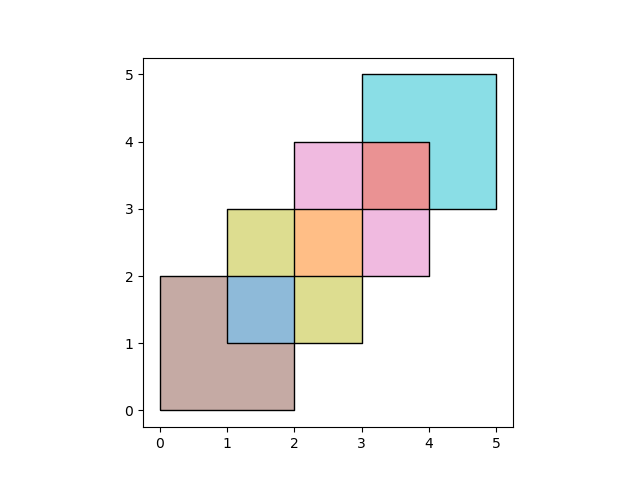The other `how` operations will return different subsets of those geometries. With `how='intersection'`, it returns only those geometries that are contained by both GeoDataFrames:

```In : res_intersection = df1.overlay(df2, how='intersection')

In : res_intersection
Out:
df1  df2                                           geometry
0    1    1  POLYGON ((2.00000 2.00000, 2.00000 1.00000, 1....
1    2    1  POLYGON ((2.00000 2.00000, 2.00000 3.00000, 3....
2    2    2  POLYGON ((4.00000 4.00000, 4.00000 3.00000, 3....

In : ax = res_intersection.plot(cmap='tab10')

In : df1.plot(ax=ax, facecolor='none', edgecolor='k');

In : df2.plot(ax=ax, facecolor='none', edgecolor='k');
```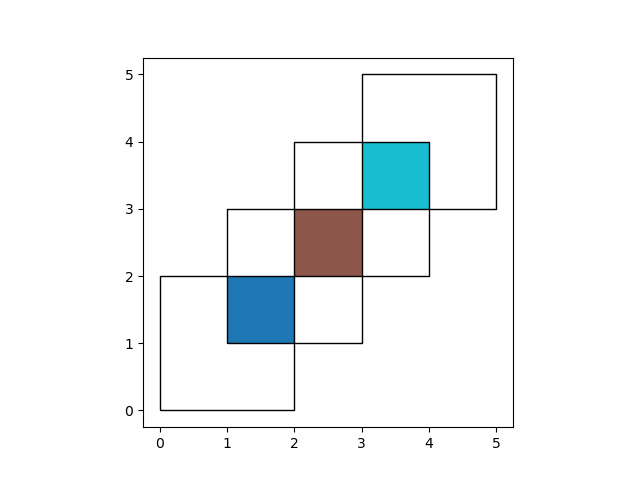`how='symmetric_difference'` is the opposite of `'intersection'` and returns the geometries that are only part of one of the GeoDataFrames but not of both:

```In : res_symdiff = df1.overlay(df2, how='symmetric_difference')

In : res_symdiff
Out:
df1  df2                                           geometry
0  1.0  NaN  POLYGON ((2.00000 0.00000, 0.00000 0.00000, 0....
1  2.0  NaN  MULTIPOLYGON (((3.00000 3.00000, 4.00000 3.000...
2  NaN  1.0  MULTIPOLYGON (((2.00000 2.00000, 3.00000 2.000...
3  NaN  2.0  POLYGON ((3.00000 5.00000, 5.00000 5.00000, 5....

In : ax = res_symdiff.plot(cmap='tab10')

In : df1.plot(ax=ax, facecolor='none', edgecolor='k');

In : df2.plot(ax=ax, facecolor='none', edgecolor='k');
```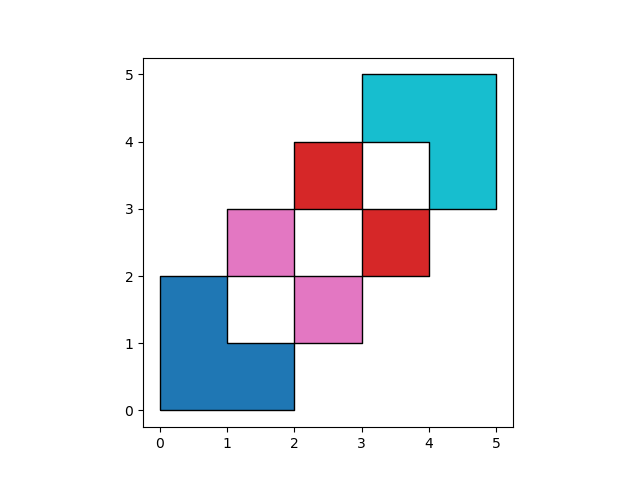To obtain the geometries that are part of `df1` but are not contained in `df2`, you can use `how='difference'`:

```In : res_difference = df1.overlay(df2, how='difference')

In : res_difference
Out:
geometry  df1
0  POLYGON ((2.00000 0.00000, 0.00000 0.00000, 0....    1
1  MULTIPOLYGON (((3.00000 3.00000, 4.00000 3.000...    2

In : ax = res_difference.plot(cmap='tab10')

In : df1.plot(ax=ax, facecolor='none', edgecolor='k');

In : df2.plot(ax=ax, facecolor='none', edgecolor='k');
```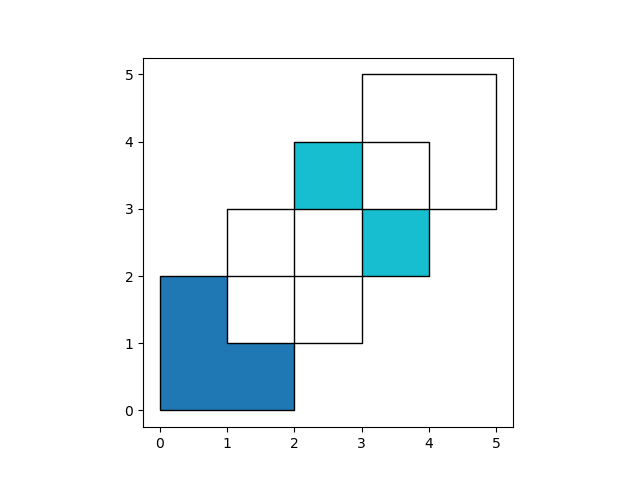Finally, with `how='identity'`, the result consists of the surface of `df1`, but with the geometries obtained from overlaying `df1` with `df2`:

```In : res_identity = df1.overlay(df2, how='identity')

In : res_identity
Out:
df1  df2                                           geometry
0  1.0  1.0  POLYGON ((2.00000 2.00000, 2.00000 1.00000, 1....
1  2.0  1.0  POLYGON ((2.00000 2.00000, 2.00000 3.00000, 3....
2  2.0  2.0  POLYGON ((4.00000 4.00000, 4.00000 3.00000, 3....
3  1.0  NaN  POLYGON ((2.00000 0.00000, 0.00000 0.00000, 0....
4  2.0  NaN  MULTIPOLYGON (((3.00000 3.00000, 4.00000 3.000...

In : ax = res_identity.plot(cmap='tab10')

In : df1.plot(ax=ax, facecolor='none', edgecolor='k');

In : df2.plot(ax=ax, facecolor='none', edgecolor='k');
```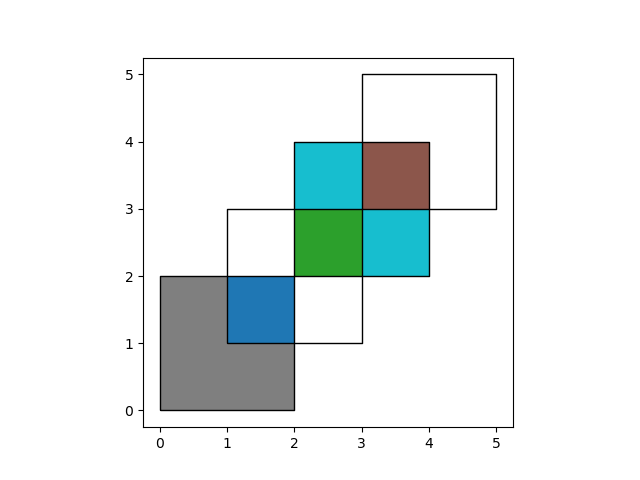## Overlay Countries Example¶

First, we load the countries and cities example datasets and select :

```In : world = geopandas.read_file(geopandas.datasets.get_path('naturalearth_lowres'))

# Select South America and some columns
In : countries = world[world['continent'] == "South America"]

In : countries = countries[['geometry', 'name']]

# Project to crs that uses meters as distance measure
In : countries = countries.to_crs('epsg:3395')

In : capitals = capitals.to_crs('epsg:3395')
```

To illustrate the `overlay()` method, consider the following case in which one wishes to identify the “core” portion of each country – defined as areas within 500km of a capital – using a `GeoDataFrame` of countries and a `GeoDataFrame` of capitals.

```# Look at countries:
In : countries.plot();

# Now buffer cities to find area within 500km.
# Check CRS -- World Mercator, units of meters.
In : capitals.crs
Out:
<Projected CRS: EPSG:3395>
Name: WGS 84 / World Mercator
Axis Info [cartesian]:
- E[east]: Easting (metre)
- N[north]: Northing (metre)
Area of Use:
- name: World between 80°S and 84°N.
- bounds: (-180.0, -80.0, 180.0, 84.0)
Coordinate Operation:
- name: World Mercator
- method: Mercator (variant A)
Datum: World Geodetic System 1984 ensemble
- Ellipsoid: WGS 84
- Prime Meridian: Greenwich

# make 500km buffer
In : capitals['geometry']= capitals.buffer(500000)

In : capitals.plot();
```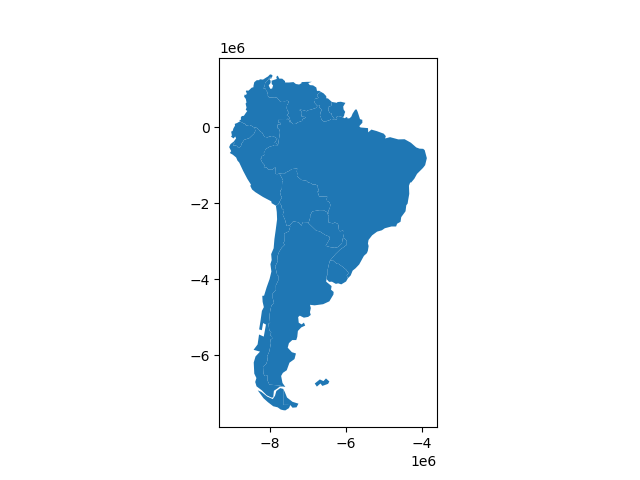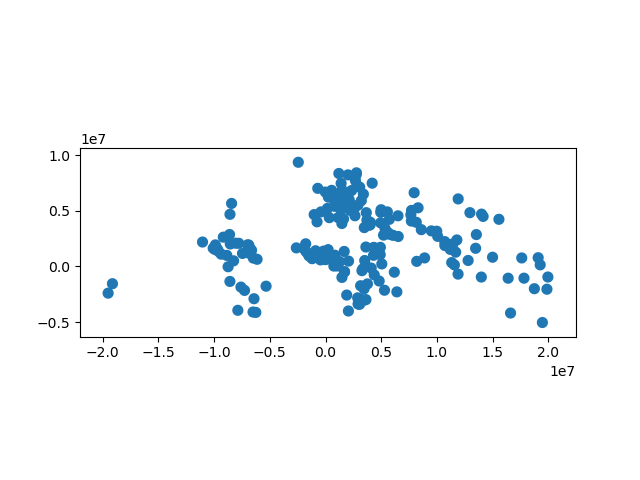To select only the portion of countries within 500km of a capital, we specify the `how` option to be “intersect”, which creates a new set of polygons where these two layers overlap:

```In : country_cores = countries.overlay(capitals, how='intersection')

In : country_cores.plot(alpha=0.5, edgecolor='k', cmap='tab10');
```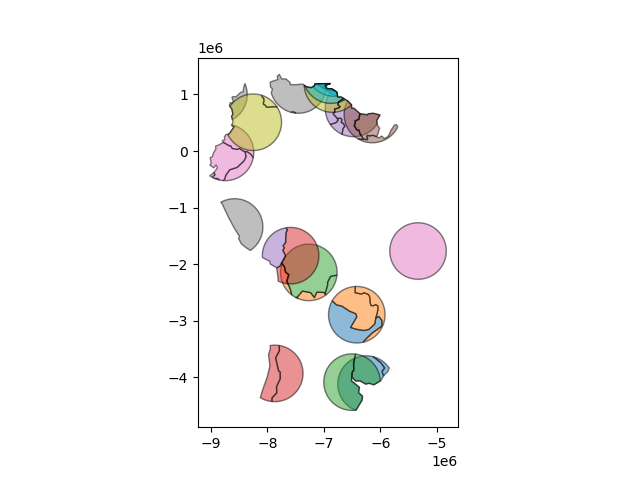Changing the “how” option allows for different types of overlay operations. For example, if we were interested in the portions of countries far from capitals (the peripheries), we would compute the difference of the two.

```In : country_peripheries = countries.overlay(capitals, how='difference')

In : country_peripheries.plot(alpha=0.5, edgecolor='k', cmap='tab10');
```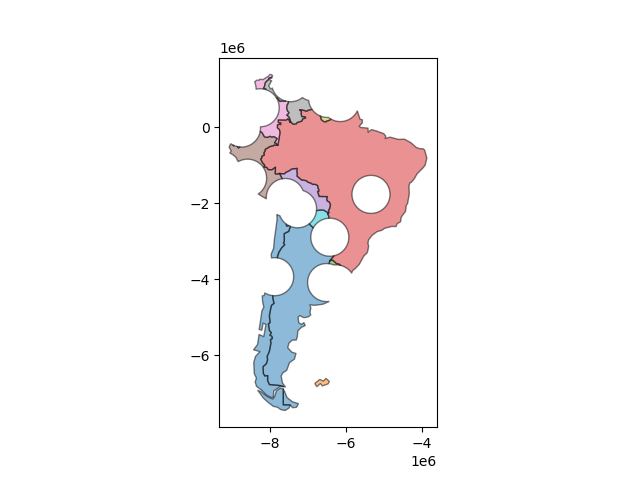## keep_geom_type keyword¶

In default settings, `overlay()` returns only geometries of the same geometry type as GeoDataFrame (left one) has, where Polygon and MultiPolygon is considered as a same type (other types likewise). You can control this behavior using `keep_geom_type` option, which is set to True by default. Once set to False, `overlay` will return all geometry types resulting from selected set-operation. Different types can result for example from intersection of touching geometries, where two polygons intersects in a line or a point.

## More Examples¶

A larger set of examples of the use of `overlay()` can be found here